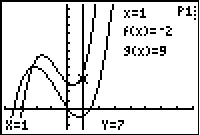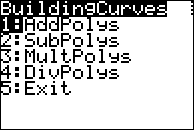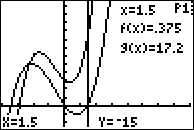••• ##### Device
• TI-83 Plus Family
• TI-84 Plus
• TI-84 Plus Silver Edition
•TI-84 Plus C Silver Edition
•TI-84 Plus CE
• ##### Software

TI Connect™
TI Connect™ CE

# Algebra II: Building curves

by Texas Instruments#### Overview

Students approach performing the basic operations on the polynomials from a graphical perspective. Given the graphs of two functions, they plot points that lie on the graph of the sum of the functions and draw conclusions about its behavior. Next, they calculate a regression to fit the points they plotted. Finally, they find the sum of the functions algebraically and compare it with the result of the regression.

#### Key Steps

•Students will build the graph of (f+g)(x) from the graphs of f(x) and g(x) by run the BLDCURVE program and choosing 1:AddPolys.

•Students will plot a point on the top curve where it intersects with the vertical line.